# Test: Triangles- 2

## 20 Questions MCQ Test Quantitative Reasoning for GMAT | Test: Triangles- 2

Description
Attempt Test: Triangles- 2 | 20 questions in 40 minutes | Mock test for GMAT preparation | Free important questions MCQ to study Quantitative Reasoning for GMAT for GMAT Exam | Download free PDF with solutions
QUESTION: 1

### In ΔLMN, LM = |x-7|, MN = |x-4| and NL = x + 1, where x is a number whose value is not known. Is ΔLMN an acute triangle (a triangle each of whose angles measures less than 90∘)? (1) NM = 10 (2) LM = 7

Solution:

Given: Some number x

• ΔLMN with sides as shown below: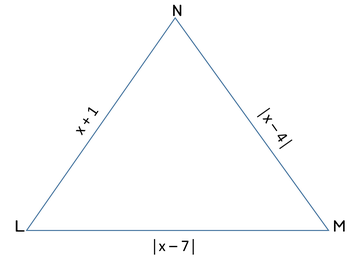Find: Is ΔLMN an acute triangle?

Step 3: Analyze Statement 1 independently

• NM = 10
• That is, |x -4| = 10
• So, x is a number whose distance from 4 on the number line is 10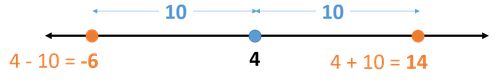• So, x = -6 or 14
• Rejecting the negative value since x + 1, being the length of a geometric figure, must be positive.
• So, x = 14
• Therefore, the sides of the triangle are:
• 15, 10, 7
• The longest side is 15
• 102 + 72 < 152
• Therefore, the triangle is obtuse.
• (Note: An obtuse triangle is a triangle one of whose angles is greater than 90)
• So, the answer to the given question is NO.
• Since we’ve been able to arrive at a unique answer to the question, Statement 1 alone is sufficient.

Step 4: Analyze Statement 2 independently

• LM = 7
• |x-7| = 7
• So, x is a number whose distance from 7 on the number line is 7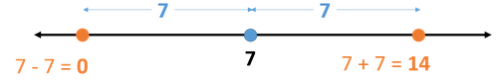• So, x = 0 or 14
• Case 1: x = 0
• The sides of the triangle are:
• 1, 4, 7
• The longest side is 7
• In this case, the sum of 2 sides of the triangle (1 + 4) is not greater than the 3rd side
• Therefore, this triangle is not possible
• So, this value of x is not possible.

• Case 2: x = 14
• So, the sides of the triangle are:
• 15, 10, 7
• The longest side is 15
• 102 + 72 < 152
• Therefore, the triangle is obtuse.
• So, the answer to the given question is NO.
• Since we’ve been able to arrive at a unique answer to the question, Statement 2 alone is sufficient
• Step 5: Analyze Both Statements Together (if needed)

Since we’ve already arrived at a unique answer in each of Steps 3 and 4, this step is not required

Hence the correct answer is Option D .

QUESTION: 2

### In ?ABC, is AC2 = AB2 + BC2? ∠BAC + ∠ACB = ∠ABC AB = BC

Solution:

Steps 1 & 2: Understand Question and Draw Inferences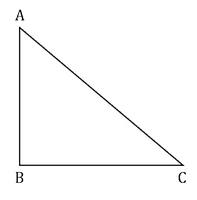Given: ?ABC

To find: Is AC2 = AB2 + BC2?

• The answer will be YES if ∠ABC=90∘,

Step 3: Analyze Statement 1 independently

Statement 1 says that ‘∠BAC + ∠ACB = ∠ABC’

• Using Angle Sum Property in ?ABC, we can write: ∠BAC+?ACB+?ABC=180∘
• Substituting the Statement 1 equation above:  ∠ABC+∠ABC=180∘
• 2(∠ABC)=180
• ∠ABC=90
• Therefore, the answer to the question is YES
• Since we’ve been able to find a unique answer to the question, Statement 1 alone is sufficient

Step 4: Analyze Statement 2 independently

Statement 2 says that AB = BC

• This means that ?ABC is isosceles
• However, not every isosceles triangle is right-angled
• Therefore, knowing only that AB = BC is clearly not sufficient to confirm that ?ABC is right-angled at B
• So, Statement 2 is not sufficient.

Step 5: Analyze Both Statements Together (if needed)

Since we’ve already arrived at a unique answer in Step 3, this step is not required

QUESTION: 3

###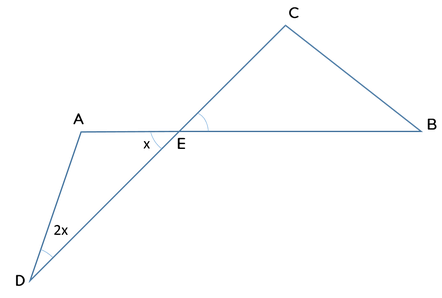Triangles AED and BEC are formed using the straight lines AB and CD as shown in the figure above. If BE = BC, DE2 > AD2 + AE2 and the measure of ∠AED is x, which of the following statements must be true? CE2 > 2BE2 AE < AD DE < CE

Solution:

Given:

• Triangles AED and BEC are formed using the straight lines AB and CD
• So, ∠AED = ∠CEB = x (Vertically opposite angles)
• In ΔBEC,
• BE = BC
• So, ∠CEB = ∠ECB = x
• Therefore, ∠EBC = 180−2x

• In ΔAED,
• We are given that DE2 > AD2 + AE2
• This means, ΔAED is obtuse
• 180−3x>90
• 90>3x
• That is, x<30∘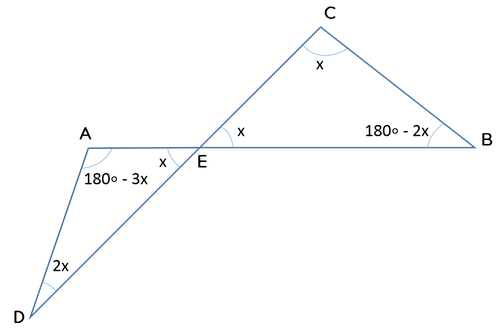To find: Which of the 3 statements must be true?

Approach:

1. Based on the given and inferred information above, we’ll evaluate the 3 statements one by one

Working Out:

• Evaluating Statement I
• Statement I says that CE2 > 2BE2
• That is, CE2 > BE2 + BC2 (since we are given that BE = BC)
• This inequality will be true if ∠EBC is obtuse.
• Checking if ∠EBC is obtuse
• ∠EBC = 180−2x
• Inferred above: x<30
• So,−2x>−60∘  (multiplying both sides with a negative number reverses the sign of inequality)
• 180–2x>180–60
• 180–2x>120
• That is, ∠EBC>120
• Since ∠EBC is indeed obtuse, Statement I will be true for all values of x

• Evaluating Statement II
• Statement II says that AE < AD
• Checking if this statement is true:
• In ΔAED, the angles in increasing order of magnitude are:
• ∠AED < ∠ADE < ∠DAE
• Note that since we’ve already inferred that ∠DAE is an obtuse angle, it will definitely be the greatest angle of this triangle. Among the remaining 2 angles, the angle that measures 2x will obviously be greater than the angle that measures x.

• So, the sides of this triangle in increasing order of magnitude will be:
• AD < AE < DE
• Thus, Statement II is NOT true.

• Evaluating Statement III
• Statement III says that DE < CE
• This inequality compares the sides of two different triangles. We do not have enough information to make this comparison. So, it is not a must be true statement based on the limited information that we have.

• Getting to the final answer
• Thus, only Statement I is a must be true statement.

Looking at the answer choices, we see that the correct answer is Option A

QUESTION: 4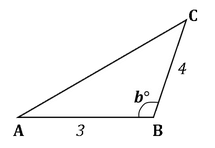The figure above shows a triangle ABC in which the measure of ∠ABC, b∘is greater than 90∘

If AB = 3 units and BC = 4 units, which of the following can be the measure of side AC?

1. 6
2. 7
3. 8

Solution:

Given:

• An obtuse triangle, 2 of whose sides are AB = 3 and BC = 4 units

To find:

• The possible measure of the side AC that is opposite to the obtuse angle

Approach & Working:

• To answer the question, we need to think of the constraints on AC. From the theory of triangles, we can figure that there are 2 constraints on AC:
• Constraint 1: Since triangle ABC is obtuse-angled at B, AC2 > AB2 + BC2
• Constraint 2: The sum of any 2 sides of a triangle must be greater than the 3rd side. So, AB + BC > AC
• So, we will work out the range of possible values of AC by applying these 2 constraints, and then see, which of the 3 given values fit this range.
• Applying Constraint 1
• AC2 > 32 + 42
• AC2 > 9 + 16
• AC2 > 25
• So, AC > 5
• Applying Constraint 2
• AB + BC > AC
• So, 3 + 4 > AC
• 7 > AC
• Rewritten as: AC < 7
• Thus, we see that 5 < AC < 7
• The only value, out of the 3 options, that satisfies this range is Option I

Thus, the correct answer is Option A

QUESTION: 5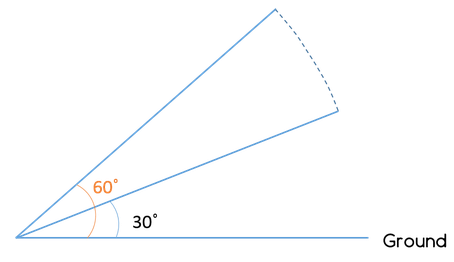The figure above shows the position, at two instants of time, of a 10-meter-long rod as it was falling down to the ground. If one end of the rod was pinned to the ground, by what vertical distance did the rod fall between the two instants of time?

Solution:

Given:

• Length of rod = 10 meters
• Angle with the ground at 1st instant = 60o
• Angle with the ground at 2nd instant = 30o

To find:

• Vertical distance covered between the 2 instants

Approach:

• We will first try to understand the question
• To do so, let us:
• Drop perpendiculars from the free end of the rod at both instants of time
• Label the triangles thus formed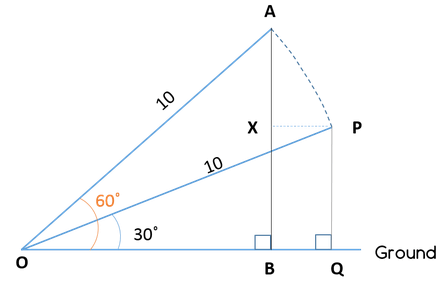• From the diagram, it is clear that we need to find AB – PQ
• (Note: if you are not sure how AB – PQ is equal to the vertical distance covered by the ladder, drop a perpendicular PX on AB. You’ll see that BQPX is a rectangle and so, PQ = BX. So, AB – PQ = AB – BX = AX. The ladder was earlier at a height of A and it is now at a height of X. So, the distance AX clearly denotes the vertical distance covered by the ladder.)
1. Thus, we need to find AB – PQ
1. AB is a side of right ΔOBA, which is 30-60- 90 Triangle and angle AOB = 60o
2. PQ is a side of right ΔOQP, which is also a 30-60-90 Triangle with angle POQ = 30o
2. In each of the 2 right triangles, we know a side and two angles.
1. So, by using side ratio property of 30-60-90 triangle, we can find the required values

Working Out:

• Finding AB
• In right ΔOBA
• OB: AB: OA = 1: √3: 2
• We know OA = 10
• AB: OA = √3: 2
• Thus AB = 5√3
• Finding PQ
• In right ΔOQP
• PQ: OQ: PO = 1: √3: 2
• We know PO = 10
• PQ: PO = 1: 2
• Thus PQ = 5
• Thus, AB – PQ = 5√3 – 5 = 5 (√3 -1) meters
• Therefore, the correct answer is Option A.
QUESTION: 6

Triangle ABC is an isosceles triangle with AB = AC and AD is the perpendicular dropped from vertex A on the side BC. What is the perimeter of triangle ABC?

(2) The perimeter of triangle ADB is 15 + 5√3

Solution:

Steps 1 & 2: Understand Question and Draw Inferences

Given:

• Isosceles ΔABC
• AB = AC
• So, perpendicular AD will bisect side BC.
• If we assume ∠ ABC to be xo, the different ∠s in the figure can be represented as below: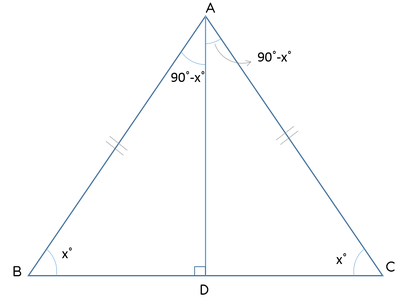To find: Perimeter of ΔABC

• =AB + BC + CA
• = 2AB + BC

So, in order to find the perimeter, we need to know AC and BC

Knowing the ∠s may help us (because we may then employ trigonometric ratios)

Step 3: Analyze Statement 1 independently

90°- x° = 2x°

⇒ 3x° = 90°

⇒ x° = 30°

But we don’t know the magnitude of any side of the triangle. So, we cannot yet use trigonometric ratios to find the sides of the triangle.

Not sufficient.

Step 4: Analyze Statement 2 independently

(2) The perimeter of triangle ADB is 15 + 5√3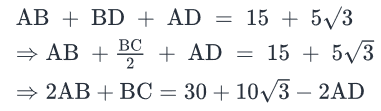The value of 2AB + BC depends on the value of AD.

Since we do not know the value of AD, we cannot find the required value

Not sufficient.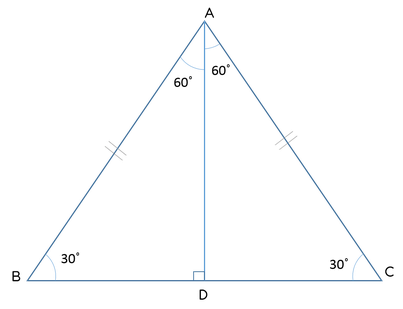• From Statement 2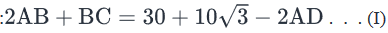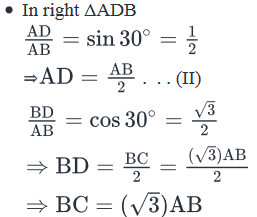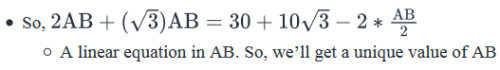• Using Equation II, a unique value of AD will be obtained
• Using Equation I, the value of 2AB + BC will be obtained

Sufficient.

QUESTION: 7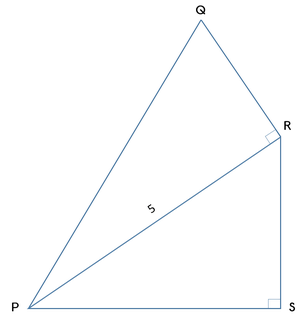The figure above shows two right triangles ΔPSR and ΔPRQ that share a common side PR, which measures 5 units. If QR = 4PS, what is the area of the quadrilateral PQRS?

(1) The area of ΔPSR is 6 square units

(2) The area of ΔPRQ is 30 square units

Solution:

Steps 1 & 2: Understand Question and Draw Inferences

Given: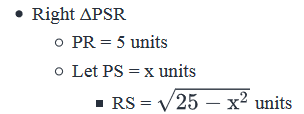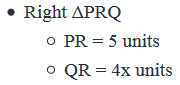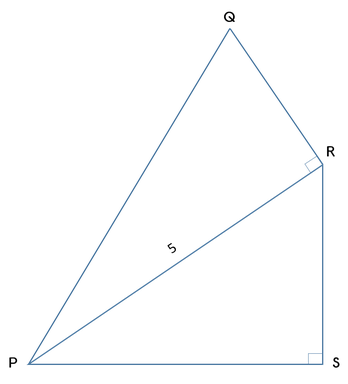To find:

• Area of quadrilateral PQRS= Area of Right ΔPSR + Area of Right ΔPRQ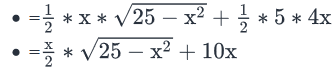So, in order to find the area, we need the value of x.

Step 3: Analyze Statement 1 independently

(1) The area of ΔPSR is 6 square units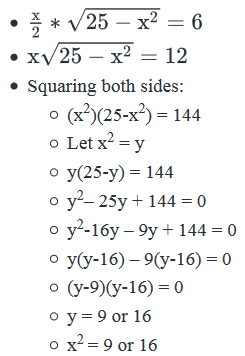• x = 3 or 4  (Rejected the negative roots because, being the length of a triangle, x cannot be negative)
• Since we didn’t get a unique value of x,
• Statement 1 is not sufficient to answer the question.

Step 4: Analyze Statement 2 independently

(2) The area of ΔPRQ is 30 square units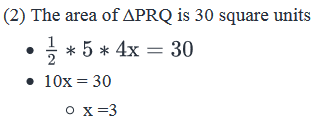• Since the value of x is unique it is sufficient to answer the question.
• Hence Statement 2 is sufficient to find the answer.

Step 5: Analyze Both Statements Together (if needed)

Since we’ve already arrived at a unique answer in Step 4, this step is not required

And the correct answer is Option B.

QUESTION: 8

Triangle ABC is inscribed in a rectangle ABEF forming two right triangles: AFC and BEC. Is triangle ABC an equilateral triangle?Solution:

Steps 1 & 2: Understand Question and Draw Inferences

Given:

The given information corresponds to the following figure: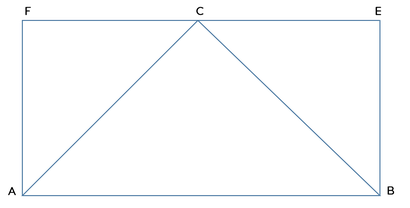To find: Is triangle ABC equilateral?

Step 3: Analyze Statement 1 independently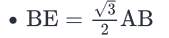• Let’s drop a perpendicular CD on side AB.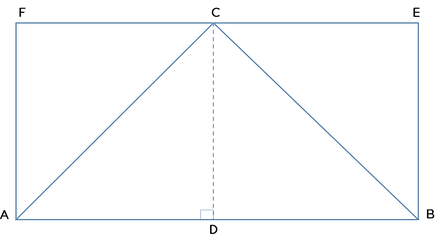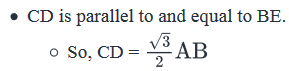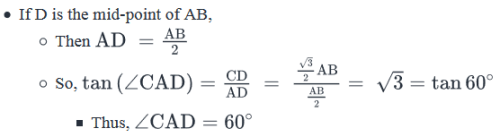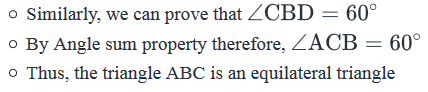• But the question is, is D the mid-point of AB?

We do not know.

Therefore, Statement 1 is not sufficient to answer the question.

Step 4: Analyze Statement 2 independently

• Point C is the midpoint of EF
• Let’s drop a perpendicular CD on side AB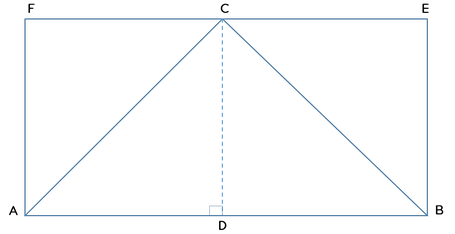• CD is parallel to and equal to BE.
• Since C is the mid-point of EF, D will be the mid-point of AB.
• But, we don’t know the magnitude of either AB or CD or AC. So, we cannot find the angles of the triangle.

Not sufficient.

Step 5: Analyze Both Statements Together (if needed)

• From Statement 1: If D is the mid-point of AB, then triangle ABC is an equilateral triangle
• From Statement 2: D is the mid-point of AB

Thus, the two statements together are sufficient to answer the question.

QUESTION: 9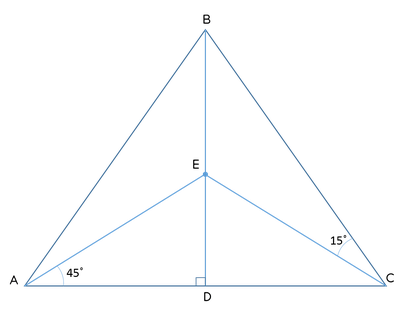In the figure above, triangle ABC is an isosceles triangle with AB = BC and BD is the perpendicular dropped from vertex B to the side AC. Point E is marked on BD such that ∠DAE = 450 and ∠ECB = 150. If the ratio of length CD: BD = 1: √3. What is the ratio of the area of triangle BEC to the area of the triangle ABC?

Solution:

Given:

• Isosceles ΔABC
• AB = BC
• BD perpendicular to AC
• Since this is an isosceles Δ, BD will also bisect AC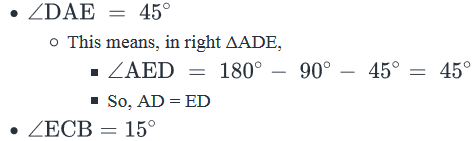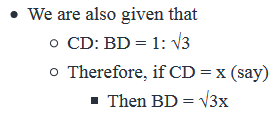• Let’s depict the given and deduced information in the diagram: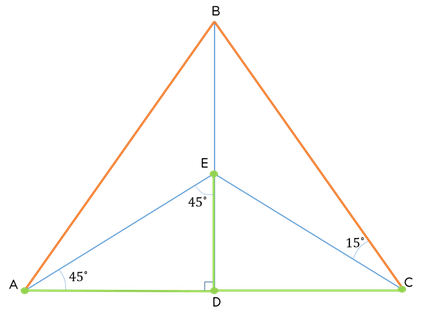• Equal sides AB and BC are depicted in orange
• Equal sides AD, ED and DC are depicted in green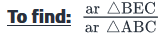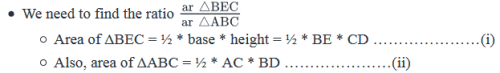We have considered: CD = x

• Since CD = ED = AD
• We can denote each of them with “x”
• Also, BD = BE + ED
• BE = BD – ED
• BE = √3x – x = (√3 – 1) x
• Also AC = AD + CD = x + x = 2x
• Substituting the value of AC, BE, BD and CD in equation (i) and (ii) we get
• Area of ΔBEC = ½ * BE * CD = ½ * (√3 – 1) x * x
• Area of ΔABC = ½ * 2x * √3x
• Thus the ratio of (Area of ΔBEC)/(Area of ΔABC) is equal to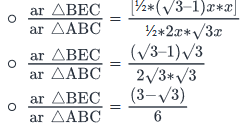Therefore, the correct answer is Option A.

QUESTION: 10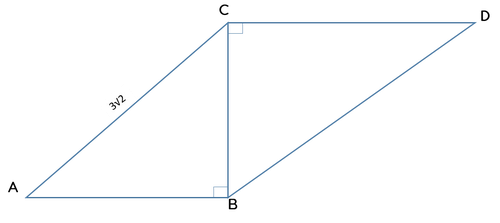In the figure above, ΔABC and ΔBCD are right-angled at points B and C respectively. If AC = 3√2, ∠ACB = 45 and ∠CBD = 30, what is the area of ΔBCD?

Solution:

Given:

• Right ΔABC
• ∠ACB = 45
• AC = 3√2

• Right ΔABC
• ∠CBD = 30
•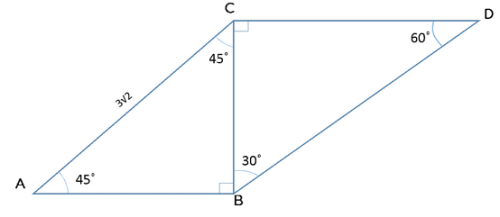To find: area of ΔBCD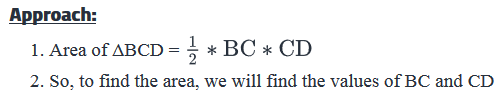Working Out:

• Finding BC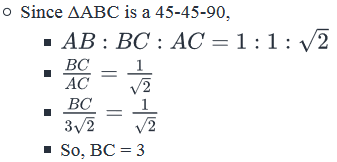Finding CD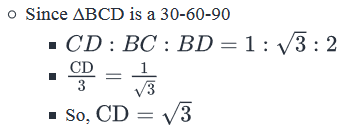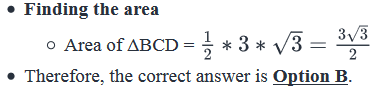QUESTION: 11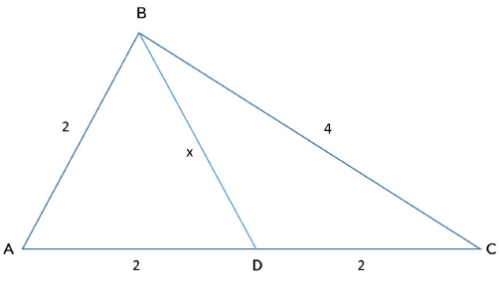In the figure shown above, x is the length of side BD of triangle ABD. If D is a point that lies on line AC and x is an integer, what is the value of x?

Solution:

Given

• In ΔABD, the sides of the triangle are: {2, 2, x}
• In ΔBDC, the sides of the triangle are: {x, 2, 4}
• x is an integer
• Clearly, since x denotes the length of a side of a triangle, x must be a positive integer.

To Find : x= ?

Approch :

• To answer the question, we need to find the constraints on x.
• Since we’re given the other side lengths of the 2 triangles, we’ll apply the property that the sum of 2 sides of a triangle is always greater than its third side, on both ΔABD and ΔBDC to get to a constraint on x.

Working out

• Applying the property in ΔABD
• 2 + 2 > x
• That is, x < 4
• And, 2 + x > 2
• That is, x > 0
• Thus, possible values of x: {1, 2, 3}

• Applying the property in ΔBDC
• 4 + 2 > x
• That is, x < 6
• And, 2 + x > 4
• That is, x > 2
• Thus, possible values of x: {3, 4, 5}
• By considering ΔABD, we got: x = {1, 2, 3}
• By considering ΔBDC, we got: x = {3, 4, 5}
• The only value of x that satisfies the property (that 2 sides of a triangle are greater than the 3 side) for both triangles is x = 3
• Therefore, the value of x is 3

Looking at the answer choices, we see that the correct answer is Option B

QUESTION: 12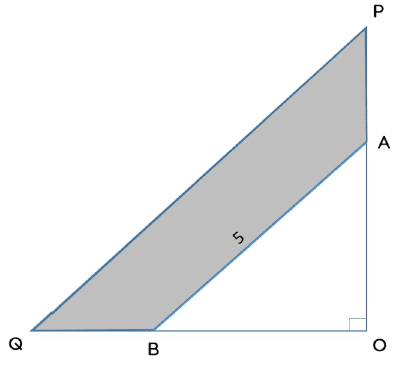In the figure above, Δ POQ and Δ AOB are both right-angled at point O. If BQ = OB and OA = AP, and the length of side AB is 5 units, what is the area of the shaded region?
(1) OA = OB + 1
(2) If a rectangle is drawn with sides of length 2QB and PA /4 the area of the rectangle will be 6 square units

Solution:

Given:
Right Δ POQ, in which:
BQ = OB
Means, B is the mid-point of OQ
OA = AP
Means, A is the mid-point of OP
AB = 5
To find:
= Area of Triangle POQ – Area of Triangle AOB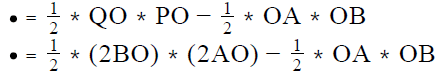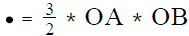So, in order to answer the question, we need to know the value of OA and OB, or at least of the product OA*OB.

Step 3 : Analyze Statement 1 independently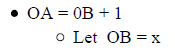• OA = x + 1
• In triangle AOB, x + x+1 = 5 (By Pythagoras Theorem)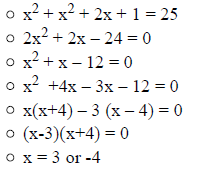Rejecting the negative value since the length of a geometric figure cannot be negative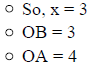Thus Statement 1 alone is sufficient to answer the question

Step 4 : Analyze Statement 2 independently
If a rectangle is drawn with sides of length 2QB and PA/4 the area of the rectangle will be 6 square units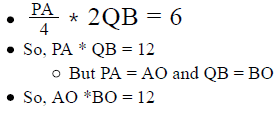Thus Statement 2 alone is also Sufficient to answer the question.

Step 5 : Analyze Both Statements together (if needed)
Since we’ve already arrived at a unique answer in each of Steps 3 and 4, this
step is not required

QUESTION: 13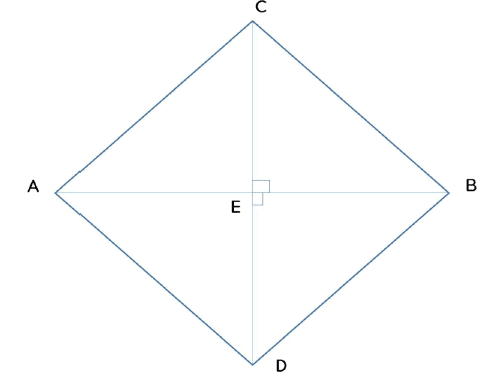In the above figure, lines AB and CD are perpendicular to one another. If AD is parallel to BC and ∠CAB = 2∠ABD, what is the
measure of ∠ACB?
(2) ∠ CBD = 60

Solution:

Steps 1 & 2: Understand Question and Draw Inferences

Given:

•     Let ∠ BAC = x°

•     So, as per the given information, the different angles in this figure will look as below:

•     In right AAEC, ∠ACE = 90 — x°(Angle Sum Property)

•     In right AAED, ∠ ADE =90 — y (Angle Sum Property)

•     Since AD is parallel to BC,

o ∠BCE = ∠ADE =90 — y° (Interior Alternate Angles)

o ∠CBA = ∠BAD = y°(Interior Alternate Angles)

•     Given: ∠CAB = 2∠ABD

That is, x° = 2∠ABD

o Therefore, ∠ ABD =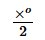O In right ABED, ∠EDB = 90° -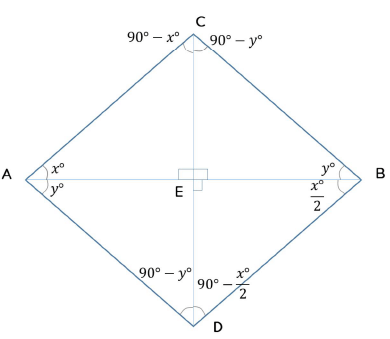To find: ∠ACB = ?

• As per the above figure, ∠ACB = 180 — (x° + y°)
• So, in order to find its value, we need to know the value of X ° + y °

Step 3: Analyze Statement 1 independently

• That is, X° + y° = 90 . Sufficient

Step 4: Analyze Statement 2 independently

• ∠ CBD = 60°
•+ y ° =   60°

Not sufficient to give us a unique value for X ° + y °.

Step 5: Analyze Both Statements Together (if needed)

Since we’ve already arrived at a unique answer in Step 3, this step is not required

QUESTION: 14

Sam climbs up a 40/√3 metres long ladder that is inclined at an angle of 60 to the ground. Upon reaching the upper edge of the ladder, he drops a coin to the ground. If the time taken, in seconds, by an object to drop by a height of h meters is equal to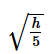, then how much time does the coin dropped by Sam take to reach the ground?

Solution:

Given

• A ladder inclined at an angle of 60 to the ground as shown below: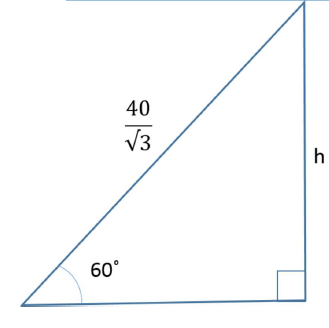Let the height from which the coin falls be h meter
Time taken to fall =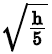seconds

To Find : Time taken to fall

Approach

• We need to find the time taken by the coin to fall
• Time taken to fall =seconds
• Since the value of time taken depends on h, we will need to find the value of h first
• The ladder, ground and the wall form a right triangle
• Since we know a side and an angle in this triangle, we can find h by using trigonometric ratios

Woeking out:

Since the right angled triangle is a 30-60-90 Triangle, we can write -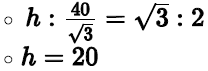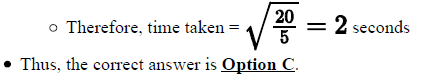QUESTION: 15

What is the value of a?
(1) The area of an equilateral triangle of side length 3a units is 9√3 square units
(2) The area of an isosceles triangle whose sides are of length 4a, 4a and a units is 3√7 square units

Solution:

Steps 1 & 2: Understand Question and Draw Inferences

To find: the value of a

Step 3: Analyze Statement 1 independently

• Area of an equilateral Δ of side length 3a is 9√3
•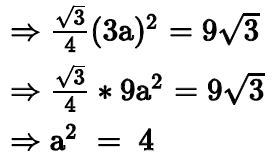So, a = 2 (rejecting a = -2 because the side of a triangle, 3a, cannot be negative)
Thus, Statement 1 alone is sufficient to answer the question

Step 4: Analyze Statement 2 independently

• The area of an isosceles triangle whose sides are of length 4a, 4a and a units is 3√7 square units
• Let’s draw the given triangle: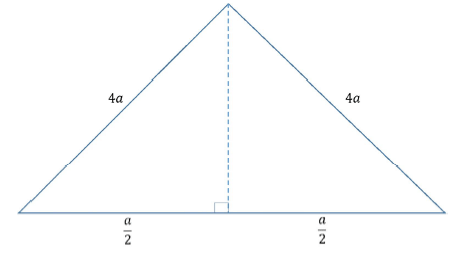• A perpendicular on the side that measures a units from the opposite vertex will bisect this side (Property of Isosceles triangles)
• Let the height of the perpendicular be h
• So, by applying Pythagoras Theorem,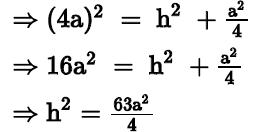=>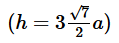(Rejecting the negative value of h since the length of a perpendicular cannot be negative)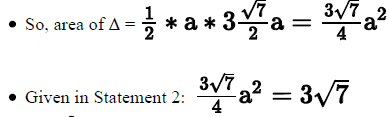so a=4

That is, a = 2 (rejecting a = -2 because the side of a triangle, 3a, cannot be
negative)

Thus, Statement 2 alone is sufficient to answer the question

Step 5: Analyze both Statements together (if needed)
Since we’ve already arrived at a unique answer in each of Steps 3 and 4, this
step is not required

QUESTION: 16

In ΔABC, point D lies on the side AB between vertices A and B.
If CD = CB, is AD > BC?
(2) ∠ACD = 30

Solution:

step 1 & 2: Understand Question and Draw Inference
Given:

Representing the given information visually as below:

• In triangle BCD, CD = CB
• So, angles opposite to these sides will be equal. Let these angles measure Xo
• Let ∠ACD = y
• So, the different angles in this figure will be as under:
•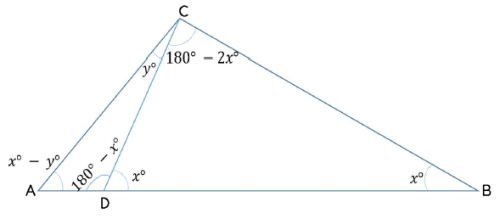To find: If AD > BC

• That is, if AD > CD (since BC = CD)
• That is, if in triangle ADC,
• ∠ACD > ∠DAC (since sides opposite to greater angles are greater)
• That is, y > x − y
• That is, 2y > x
• So, the answer to the question will be YES if
• 2y > x

​Step 3 : Analyze Statement 1 independent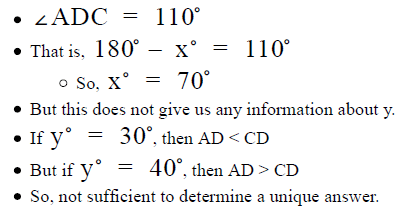Step 4 : Analyze Statement 2 independent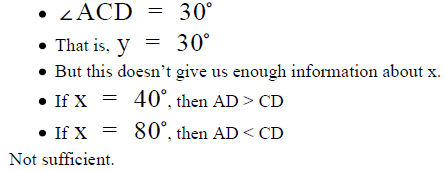Step 5: Analyze Both Statements Together (if needed)

From Statement 1:x = 70

From Statement 2: y = 30

Since we now know the values of xo and yo  we can determine if 2y> Xo

Thus, the 2 statements together are sufficient to answer the question.

QUESTION: 17

If ABC and PQR are similar triangles in which ∠ A = 470 and ∠ Q = 830, then ∠ C is: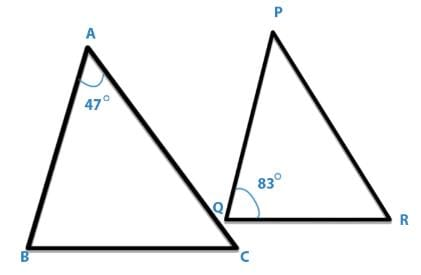Solution:

Since, Δ ABC and Δ PQR are similar triangles.
then,∠ B = ∠ Q = 830
Thus, in Δ ABC,
∠ C = 1800 - (∠ A + ∠ B)
or, ∠ C = 1800 - (470 + 830)
∠ C = 500.

QUESTION: 18

In the following figure which of the following statements is true?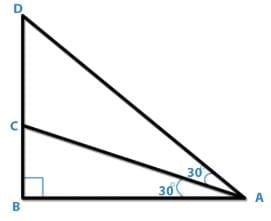Solution:

In Triangle ABD,
∠BAD + ∠B +900 = 1800
or, ∠BAD + ∠ B = 900 ------- (1)

Now, in Triangle ABC,
∠ACB + ∠B + ∠A = 1800
∠ACB + ∠B = 1800 - 900
∠ACB = 900 - ∠B -----(2)
From (1) and (2) , ∠BAD = ∠ACB
So, AC = CD.

QUESTION: 19

In triangle PQR length of the side QR is less than twice the length of the side PQ by 2 cm. Length of the side PR exceeds the length of the side PQ by 10 cm. The perimeter is 40 cm. The length of the smallest side of the triangle PQR is :

Solution:

In Δ PQR,
QR +2 = 2PQ
QR = 2PQ - 2 ------- (2)
PR = PQ + 10 ----- (2)
Perimeter = 40 m
PQ + QR + Rp = 40
Putting the value of PQ and QR from equation (1) and (2),
Pq + 2PQ - 2 + PQ +10 = 40
4PQ = 32
PQ = 8 cm which is the smallest side of the triangle.

QUESTION: 20

AB and CD bisect each other at O. If AD = 6 cm. Then BC is :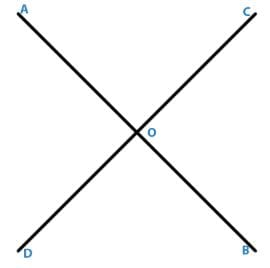Solution:

Since, AB and CD bisects each other at O,
Hence, BC = AD = 6 cm.Use Code STAYHOME200 and get INR 200 additional OFF Use Coupon Code